# Ten Frame Template PrintableApr 06, 2009FrameBy PrintableeNo Comment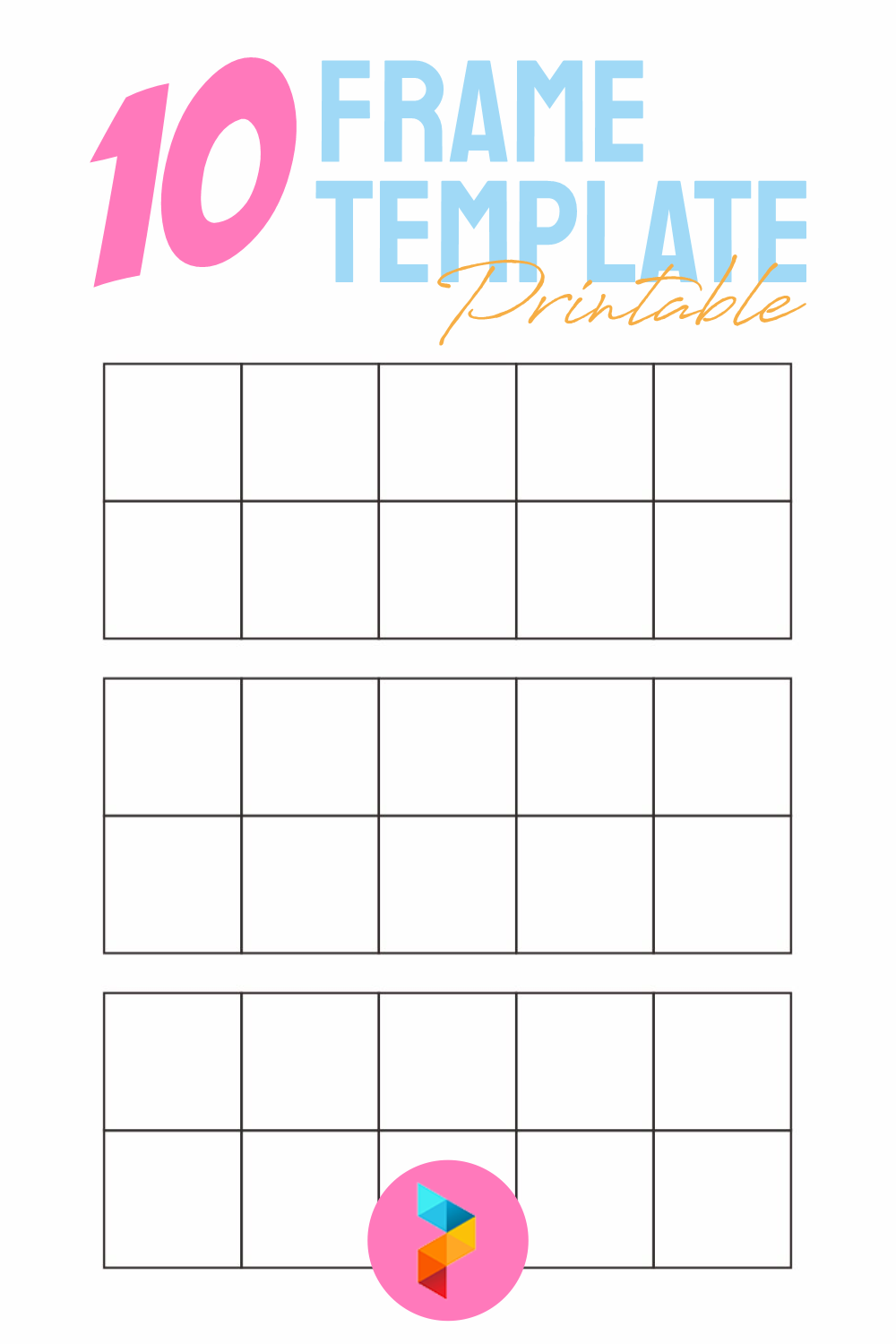Ten Frame Template Printable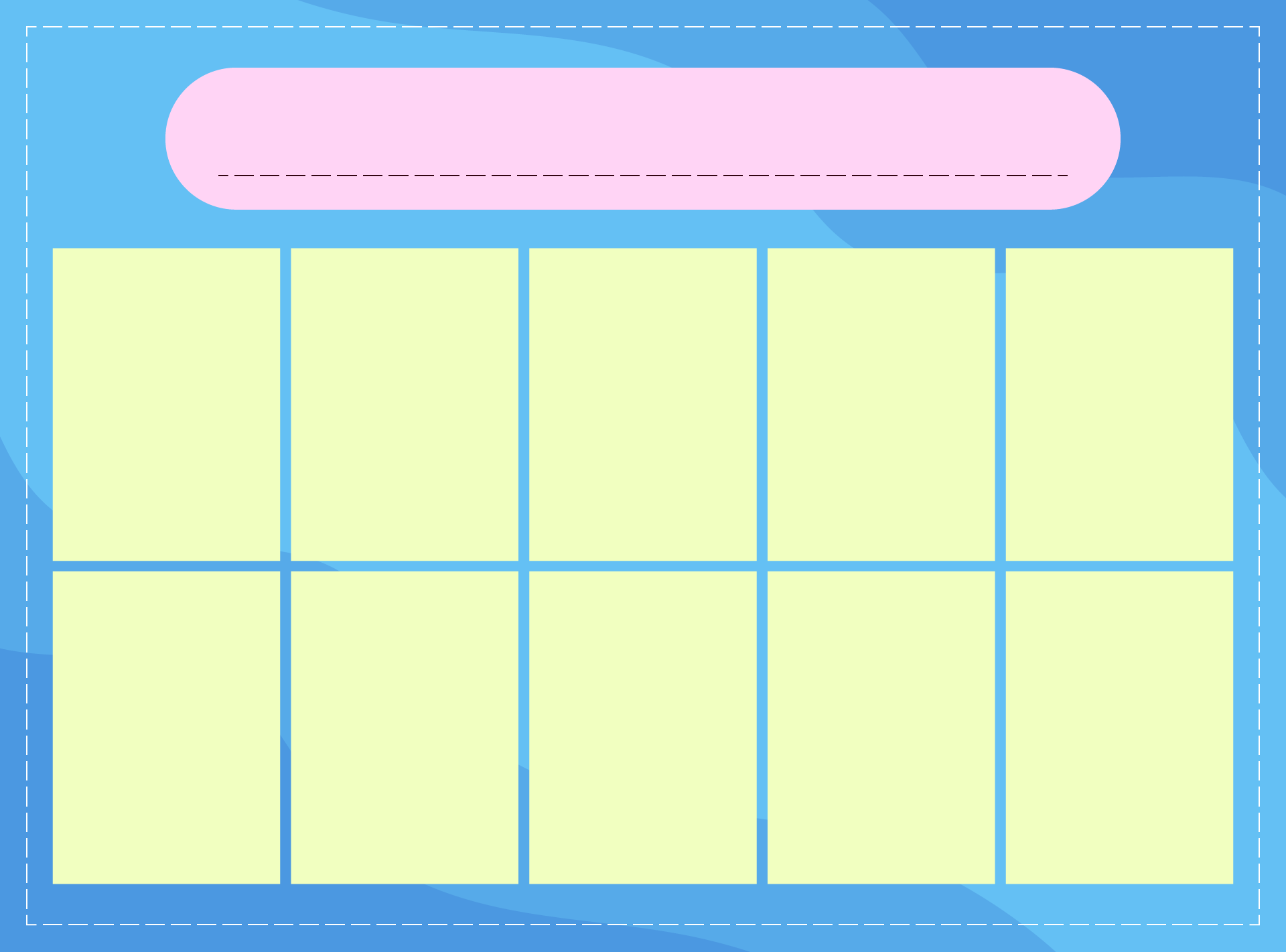### How do you use a 10 frame?

The 10 frames method is used to teach basic mathematics to children. You can teach addition and subtraction of numbers with 10 frames. The contents of 10 frames are the key. You can fill these 10 frames with dots or other interesting icons such as images of apples or stars. By using 10 frames, children can more easily understand the origin of the results of addition or subtraction. Mathematics which is usually considered a difficult problem by children will be more simple to do.

### What does a ten frame look like?

Ten frames are in the form of 2 rectangles which are divided into 10 equal parts. The rectangle turns into an equilateral square. You can make 10 frames in horizontal and vertical shapes. Take it easy, it will not affect anything because the 10 frames have not been filled with the material to add or subtract. Because the number of frames is 10, the calculation applied to 10 frames is of a scale of 10. Therefore, 10 frames are more often used by pre-school and kindergarten children. Because the questions that need to be done are still at a very easy level. It called as tasks to introduce mathematics.

### How do ten frames help students?

Without realizing it by children, using the 10 frames method can stimulate their senses towards numbers. It is easier for children to remember because the calculation on a scale of 10 is the most basic and round number calculation. Children can develop the number 10 in the form of other calculations on a scale of 10 and use 10 frames. Most importantly children become familiar first, using ten frames in their learning and play activities. By involving 10 frames in their activities, children will see for themselves how the 10 frames work best for them to solve the problem.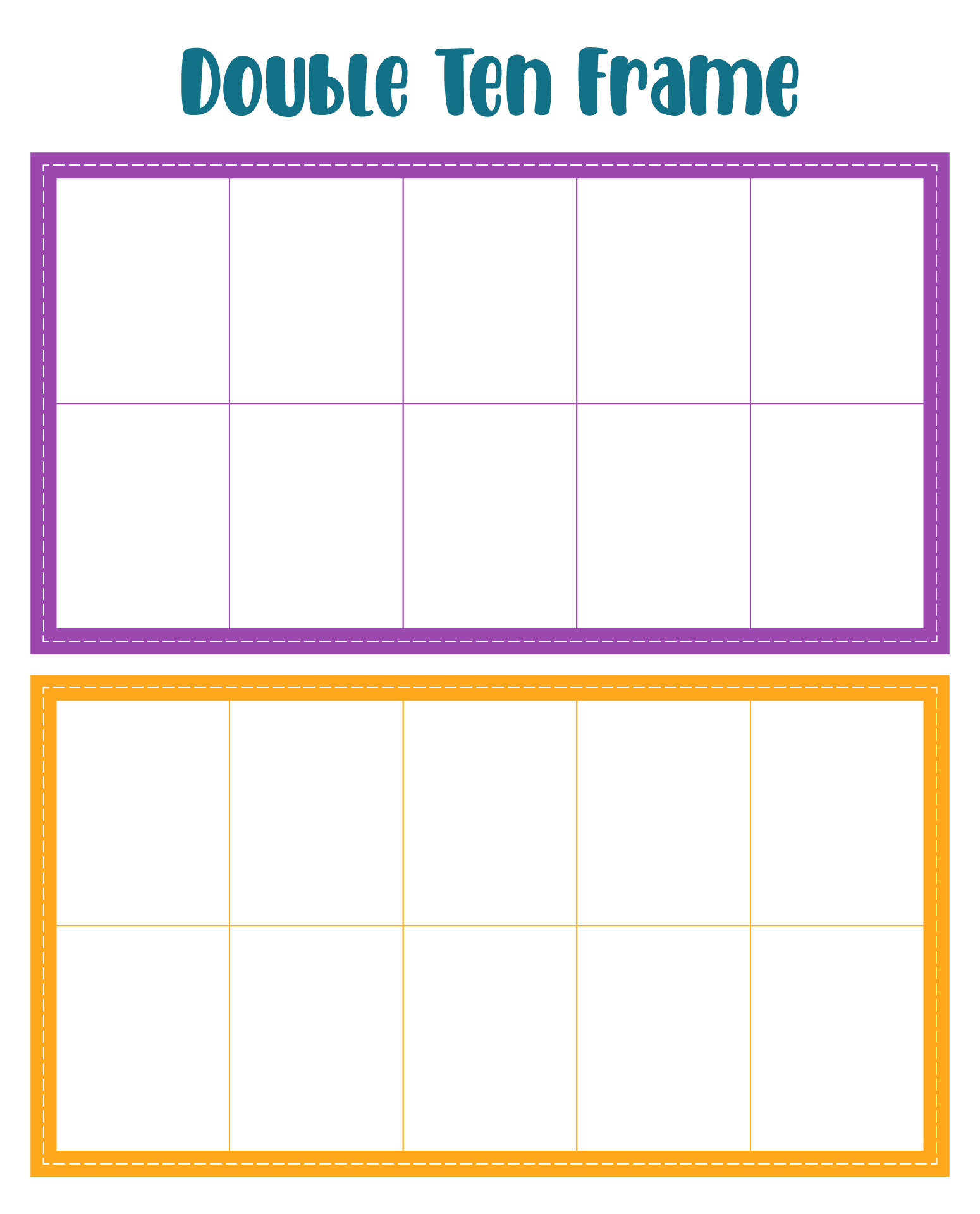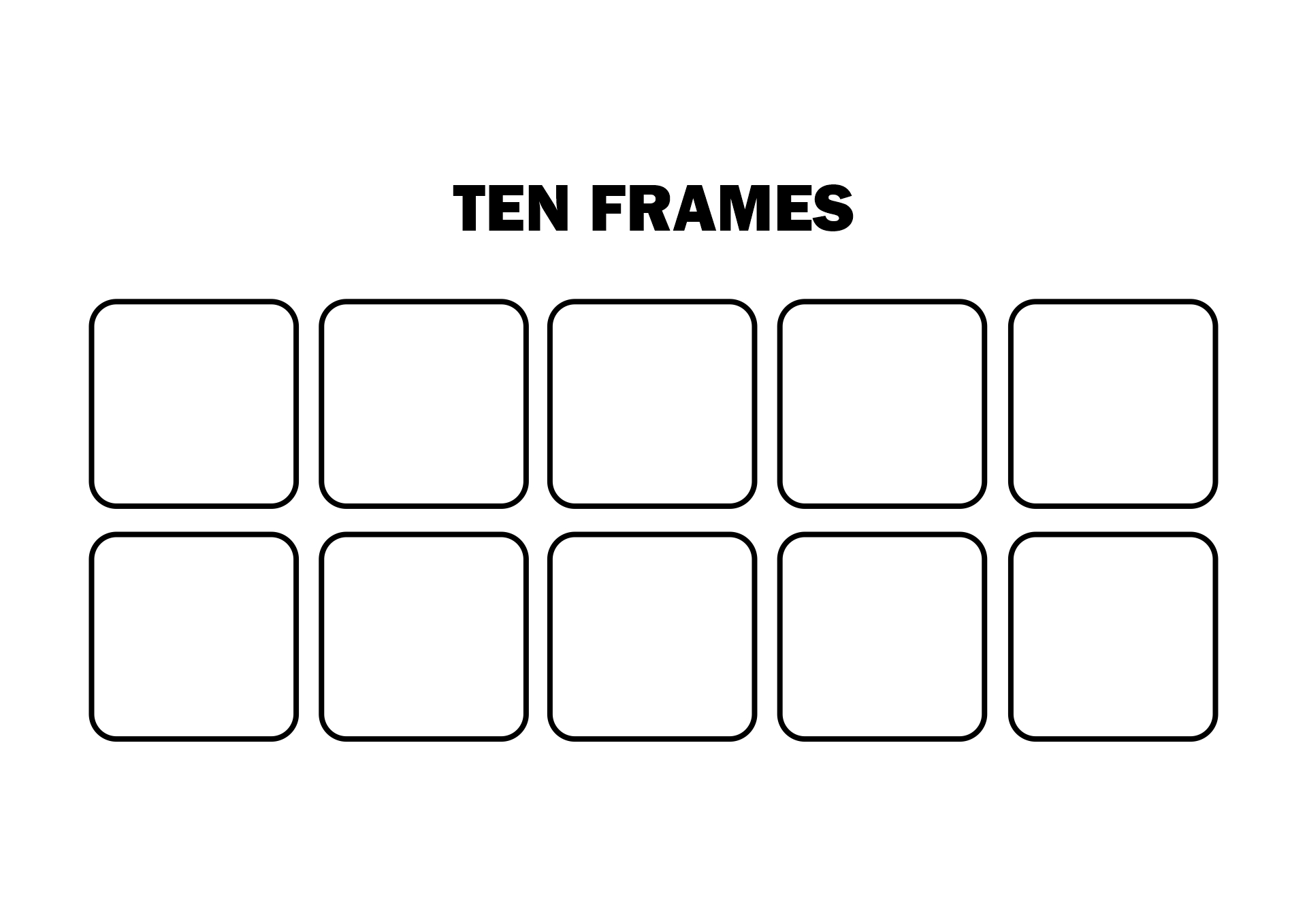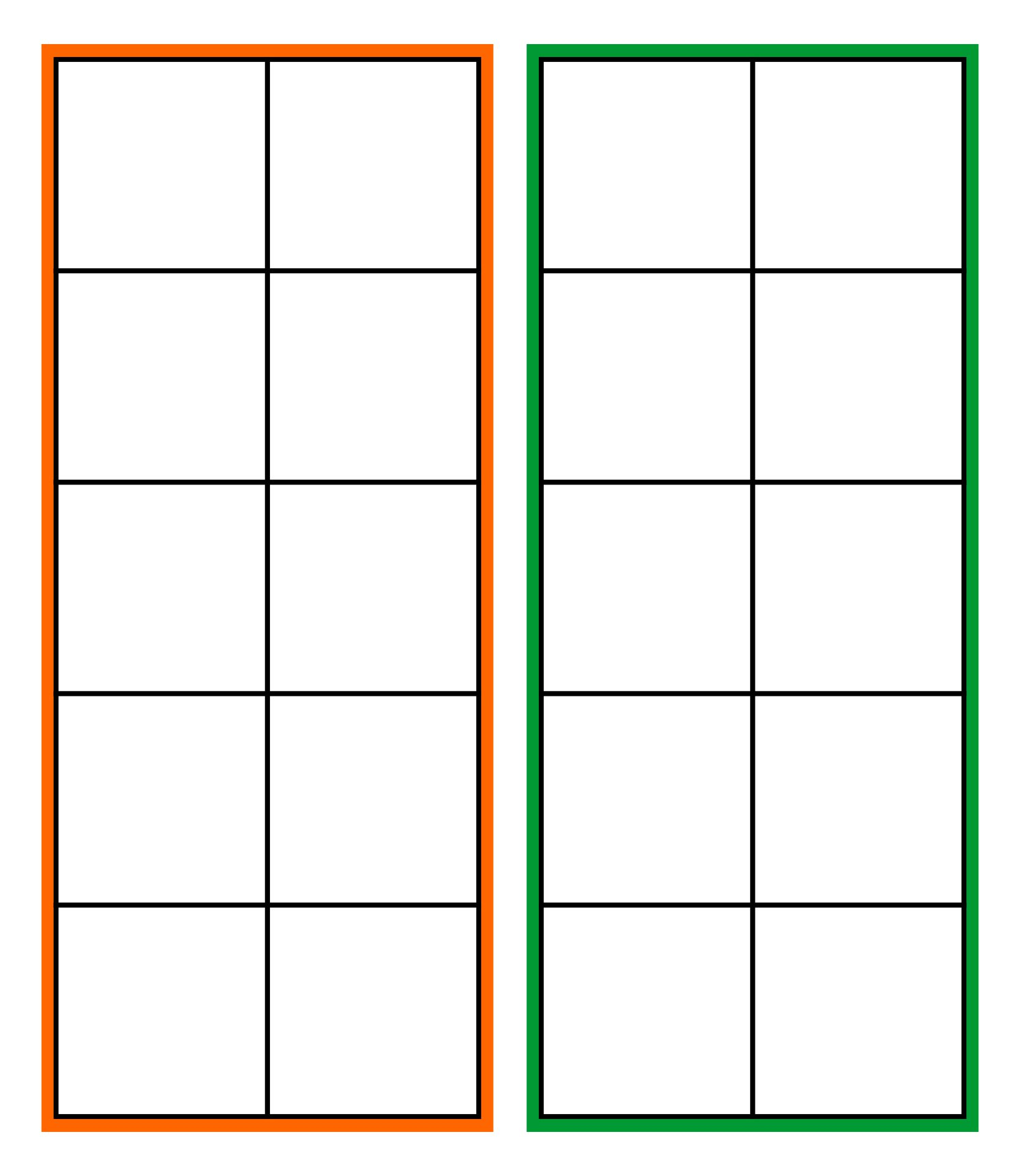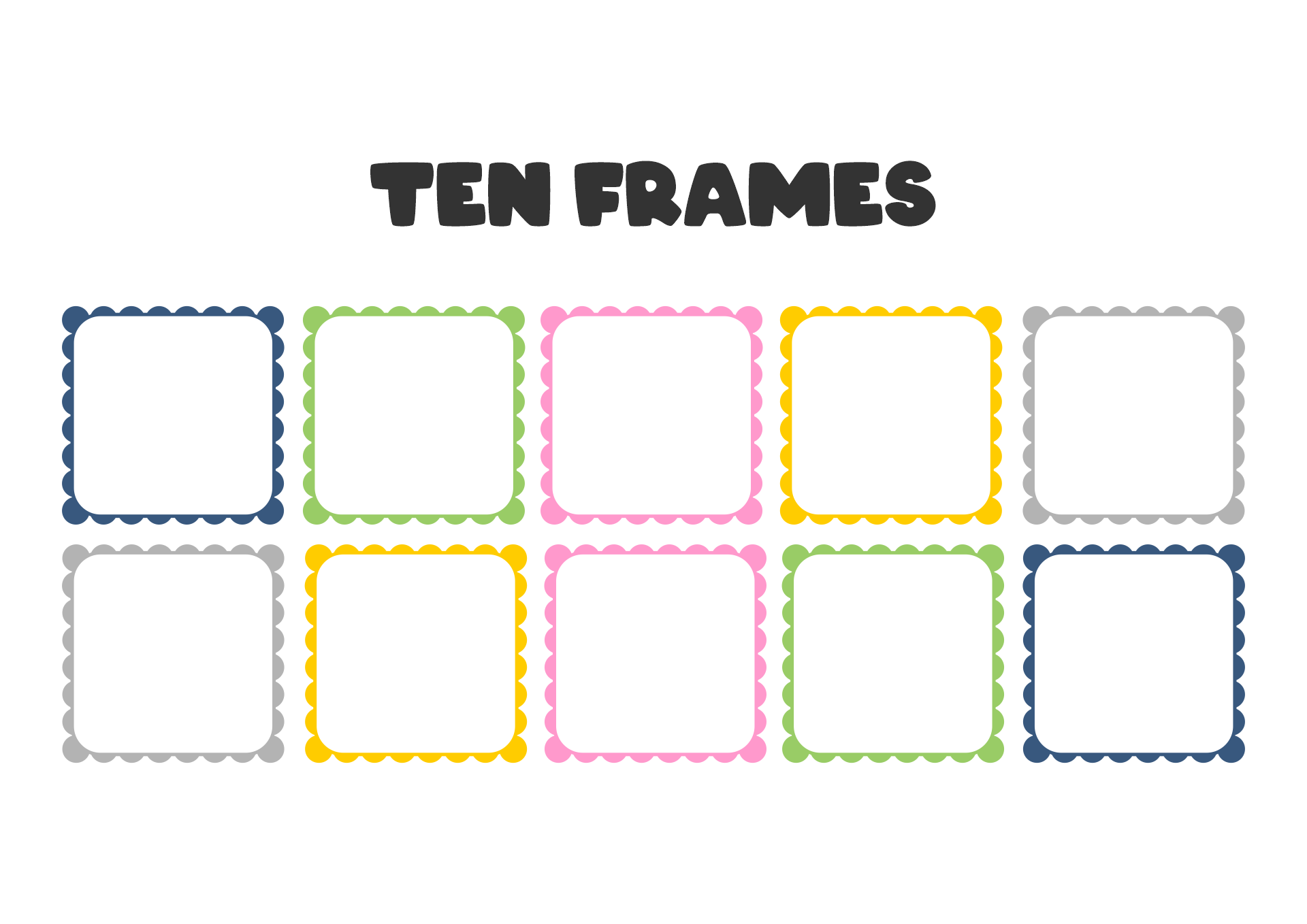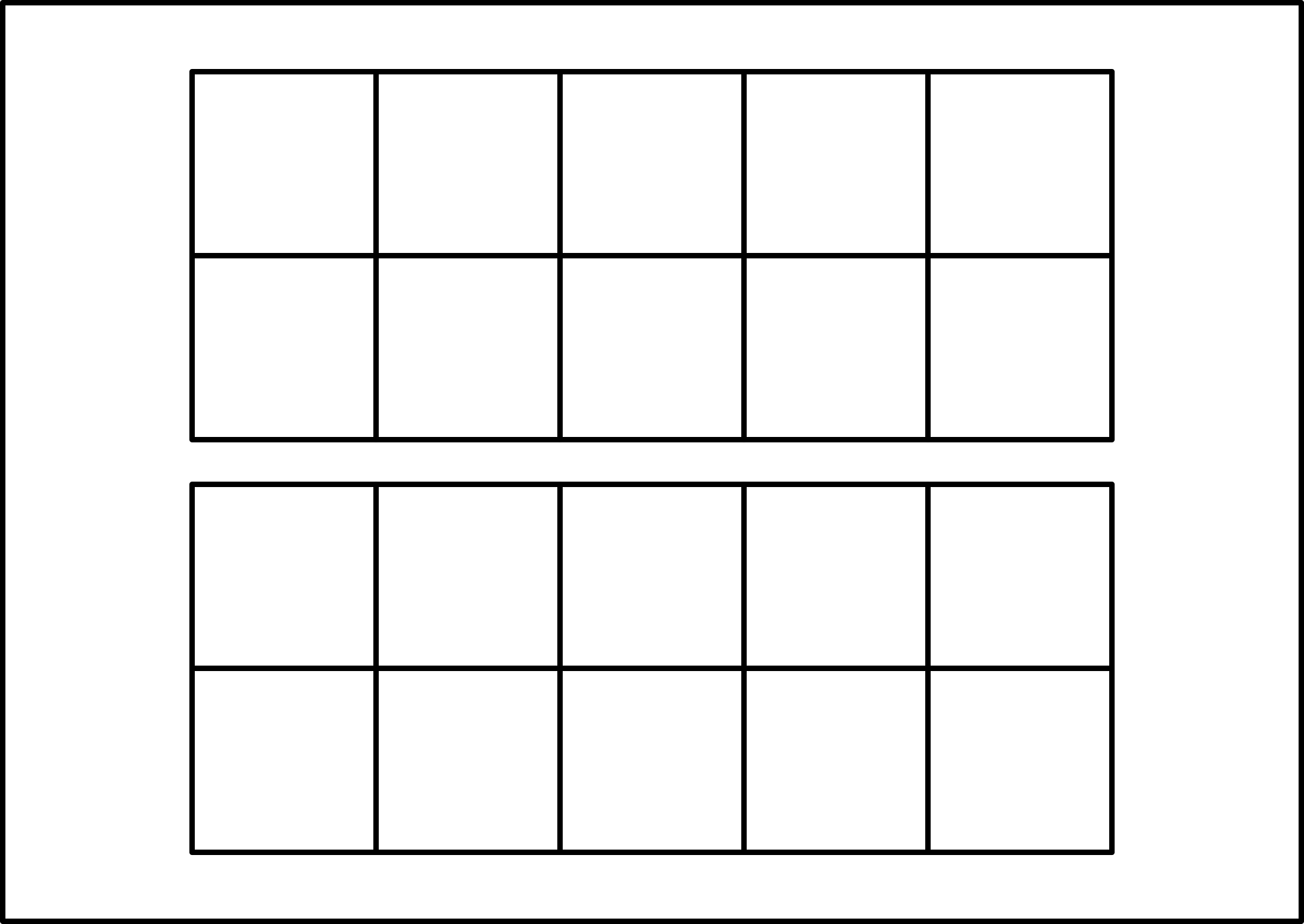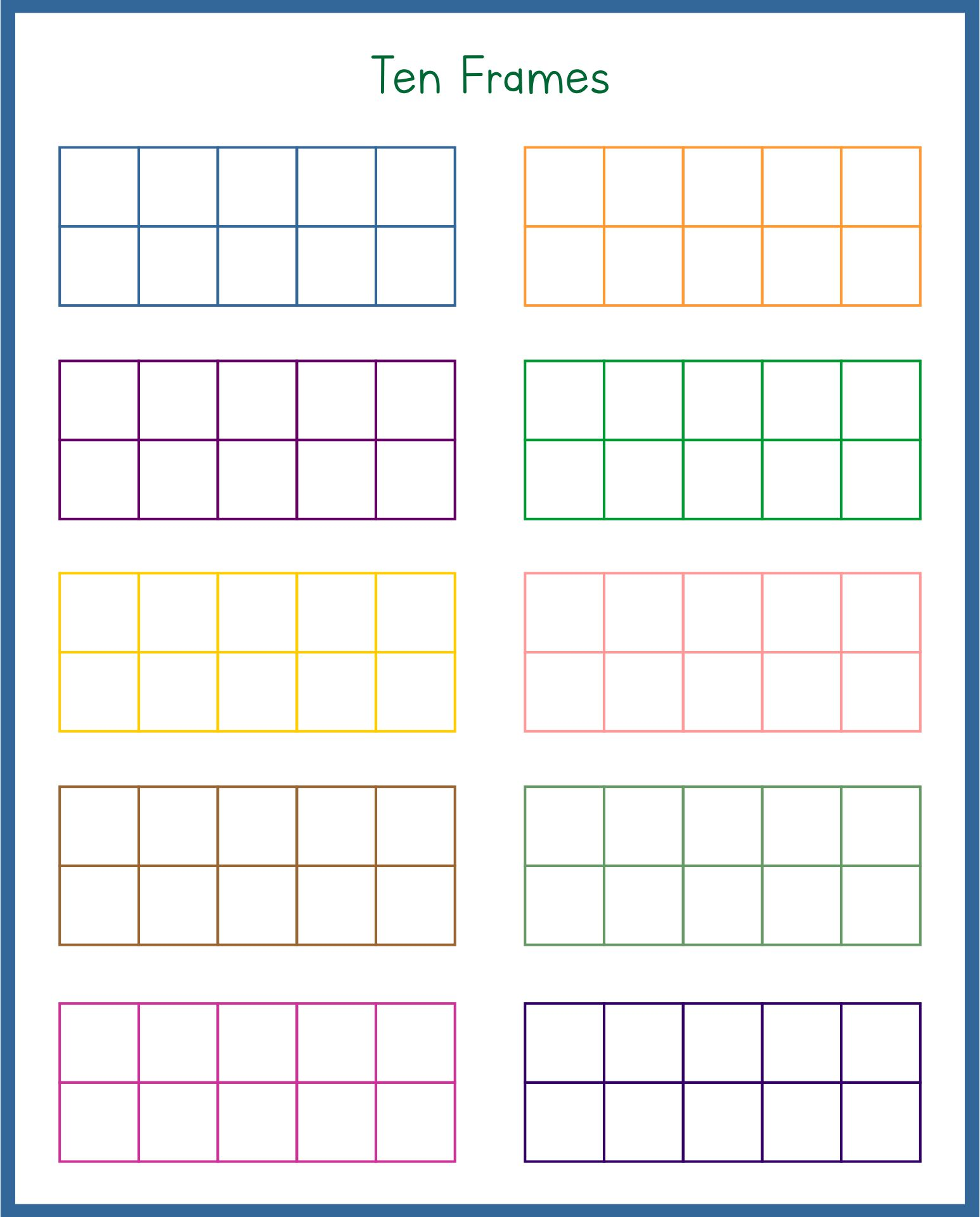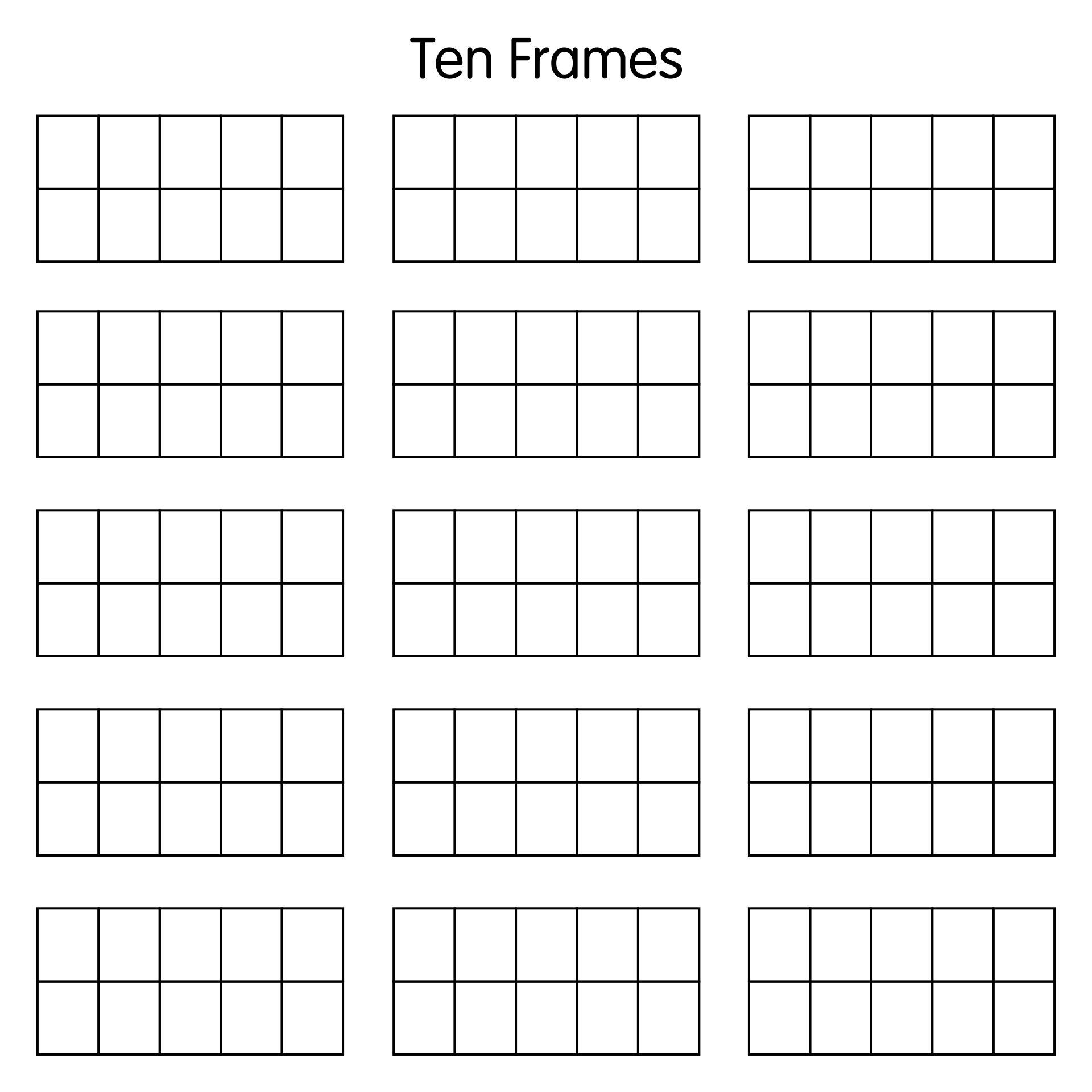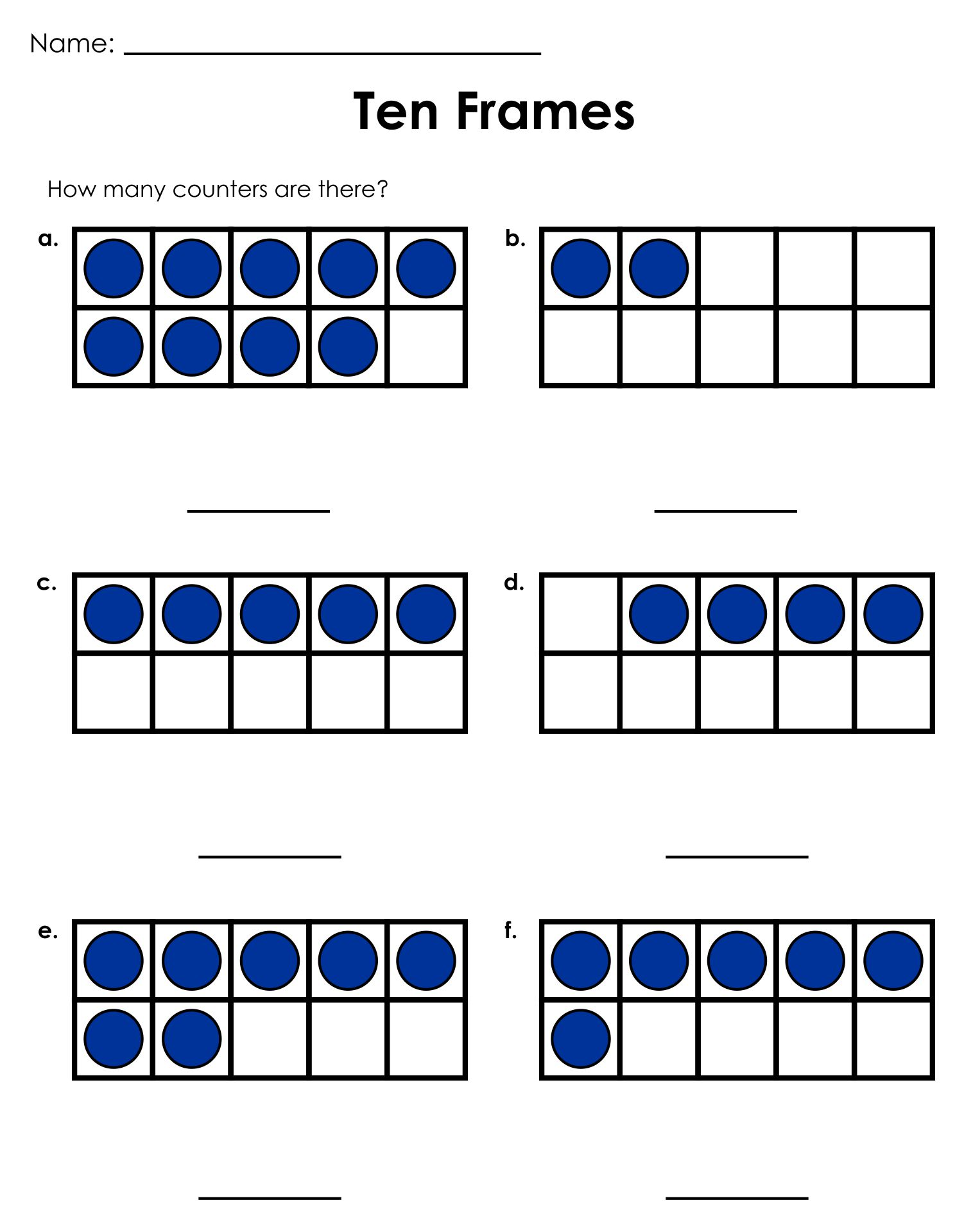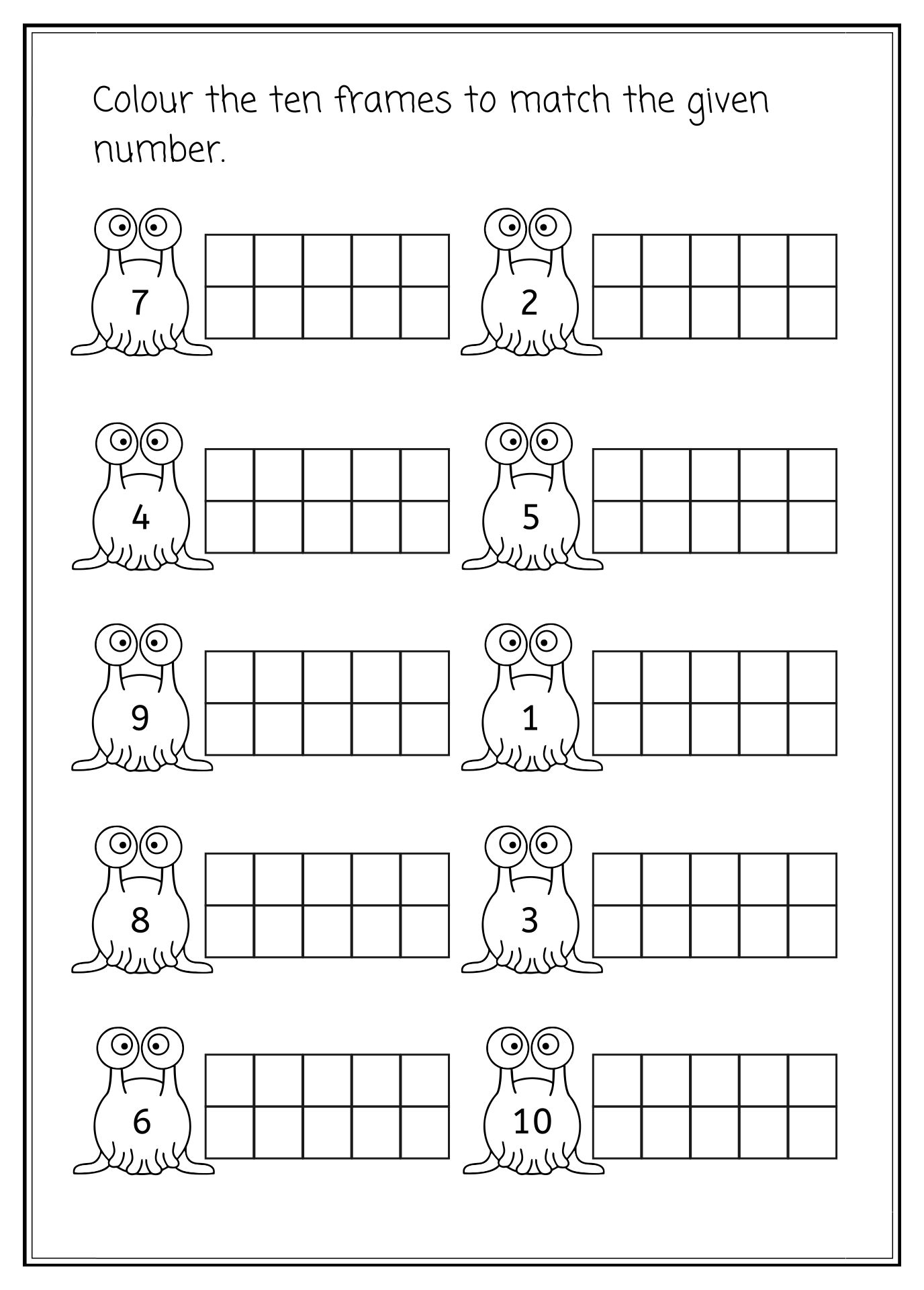### How do you make a 10 to add using ten frames?

Let's do a basic math example using 10 frames. This is very easy. To be more fun, give the children questions about the form of stories. You can put questions in the form of stories outside the frames. It can be above or below the frames. An example of the story is as follows: You have 1 apple. Then you give it to a child who already has an apple. How many apples does a child have? To solve it, ask the child to draw one apple in one frame. Explain it's the apple they have. Ask them to draw 1 more apple in the next frame. Then, explain that all the apples in the frame are the answer to the problem.

#### More printable images tagged with:

You May Like